# Low-pass filter

A low-pass filter removes all frequencies above the cutoff frequency. This frequency is determined by the values of the components.

### RC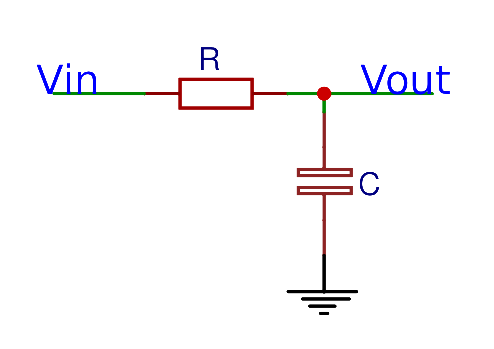#### Formulas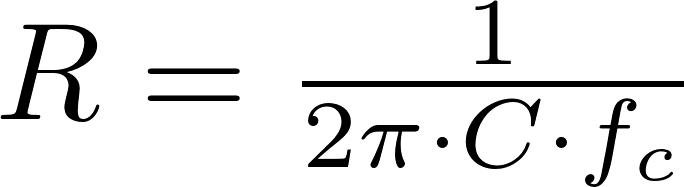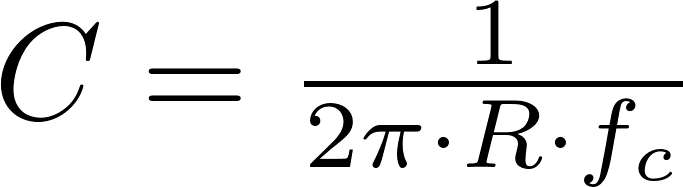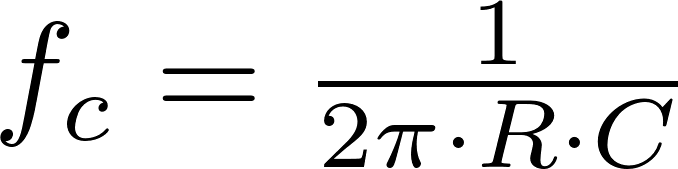R is the symbol for resistance and is measured in ohm (Ω).
C is the symbol for capacitance and is measured in farad (F).
f c is the symbol for cutoff frequency and is measured in hertz (Hz).

### RL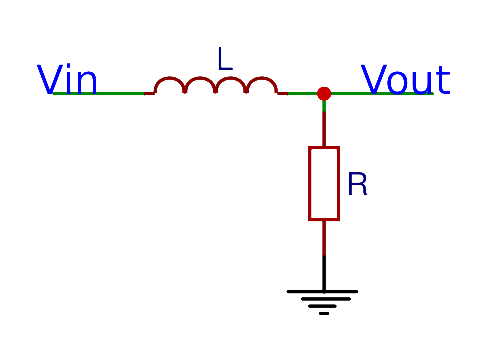#### Formulas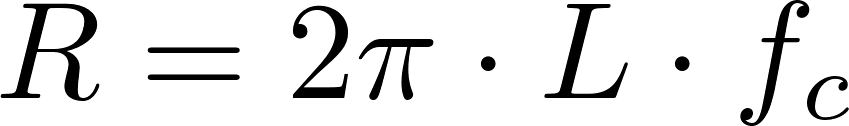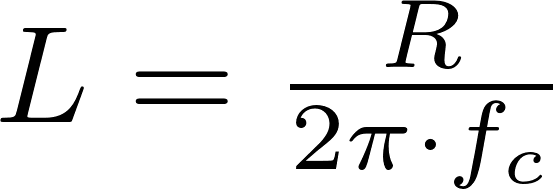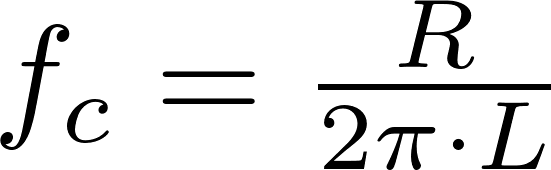R is the symbol for resistance and is measured in ohm (Ω).
L is the symbol for inductance and is measured in henry (H).
f c is the symbol for cutoff frequency and is measured in hertz (Hz).

### RLC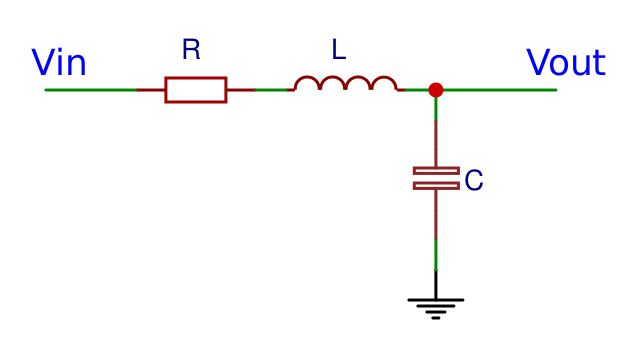#### Formulas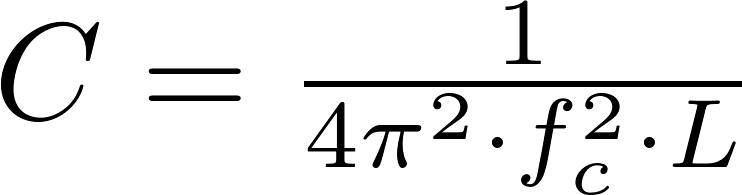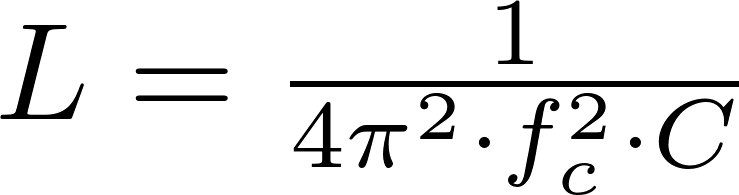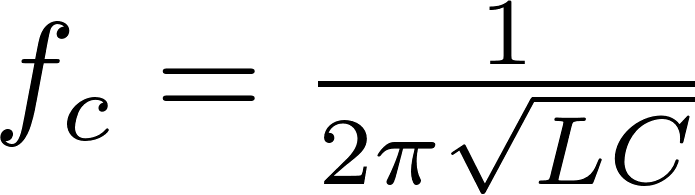C is the symbol for capacitance and is measured in farad (F).
L is the symbol for inductance and is measured in henry (H).
f c is the symbol for cutoff frequency and is measured in hertz (Hz).